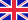•# The Effect of Heat Load on Fluid Friction Factor in Corrugated Hoses

Autor: Peter Romeo Nyarko

In this book, the effect of heat load on fluid friction factor for laminar flow of a 2D axisymmetric straight pipe is analyzed. Some clever assumptions are made on the Navier-Stokes equations to derive analytic expressions for computing the friction factor... Viac o knihe

Na objednávku, dodanie 2-4 týždne

42.84 €

bežná cena: 50.40 €

## O knihe

In this book, the effect of heat load on fluid friction factor for laminar flow of a 2D axisymmetric straight pipe is analyzed. Some clever assumptions are made on the Navier-Stokes equations to derive analytic expressions for computing the friction factor for fluid flow in a corrugated pipe in terms of average velocity, pressure drop and the discharge of fluid. The coupled momentum and energy equations are simultaneously solved numerically using the finite element package Comsol Multiphysics. A theoretical velocity profile is derived for fluid flow in a pipe with arbitrary number of layers as a result of the heat load on the pipe wall. A new geometry for computing the friction factor in fluid flow that takes care of the instabilities in the solution in a periodically corrugated pipe is developed in Comsol Multiphysics. It is shown numerically that heat load on fluid flow decreases the friction factor in corrugated hoses. Finally we introduce bubbles into the flow and study its effects on the friction factor.

• Vydavateľstvo: LAP LAMBERT Academic Publishing
• Rok vydania: 2011
• Formát: Paperback
• Rozmer: 220 x 150 mm
• Jazyk: Anglický jazyk
• ISBN: 9783846549957

Generuje redakčný systém BUXUS CMS spoločnosti ui42.